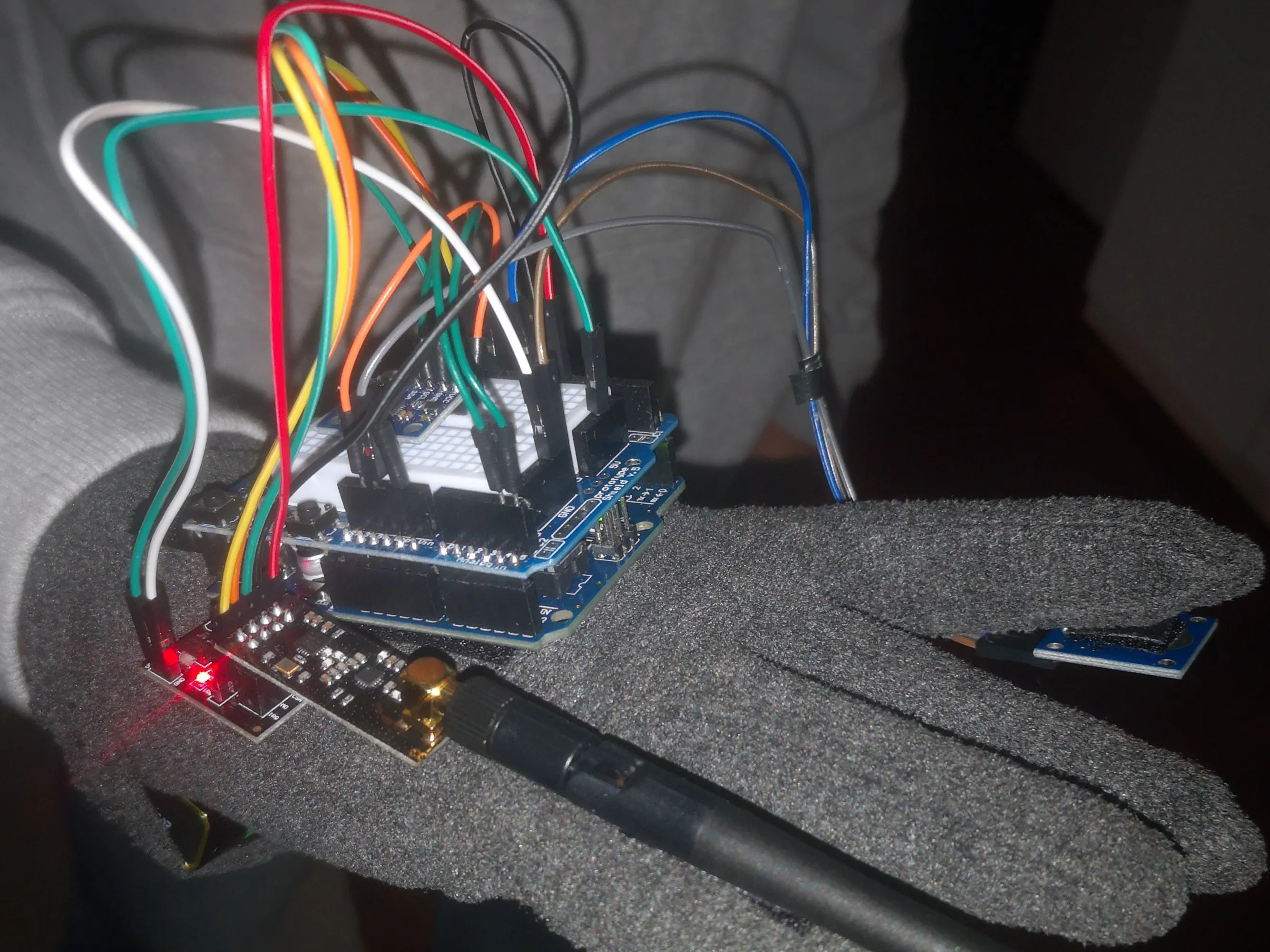# Gesture Glove © TAPR-OHL

From today you can say goodbye to mouses, thanks to the new Gesture Glove, which is able to replace all his function!

• 3,656 views
• 0 comments
• 9 respects

## Components and supplies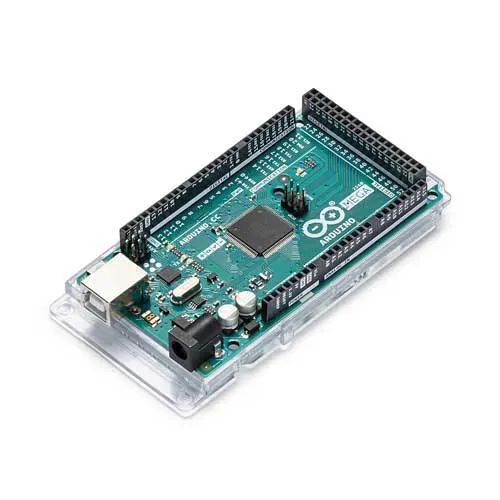Arduino Mega 2560
×1Arduino UNO
×1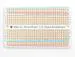Perma-Proto Breadboard Half Size
×1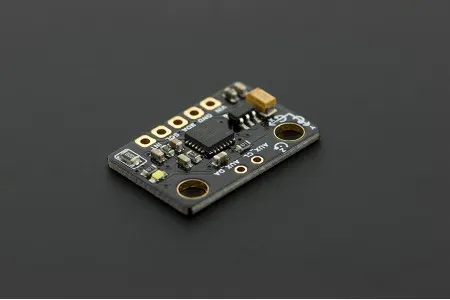DFRobot 6 DOF Sensor - MPU6050
×1
 nRF24 Module (Generic)
×2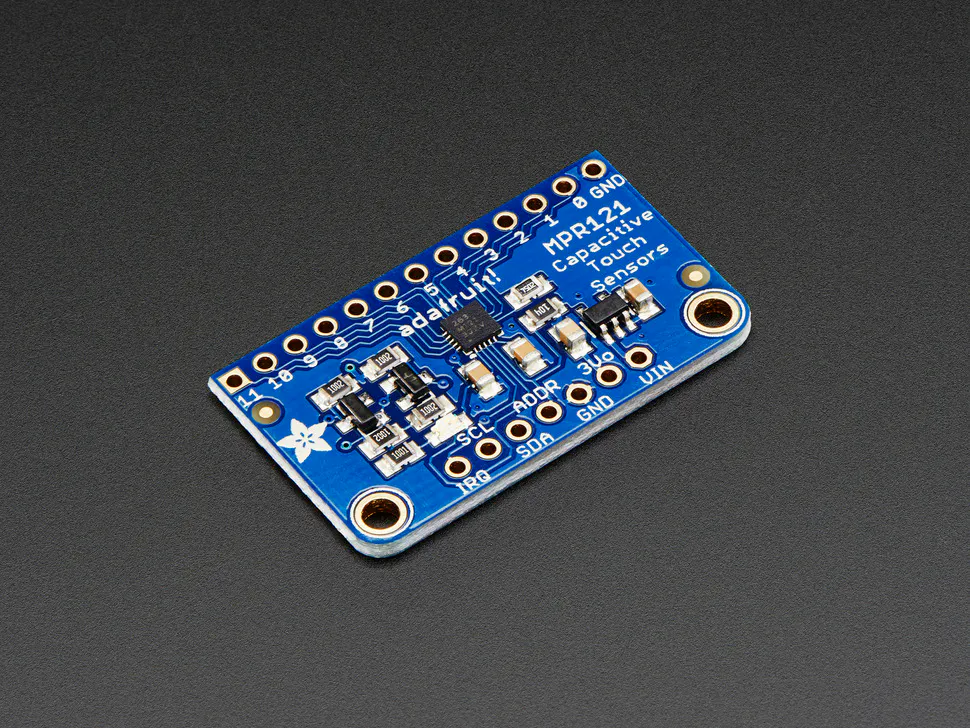Adafruit Capacitive Touch Sensor Breakout - MPR121
×1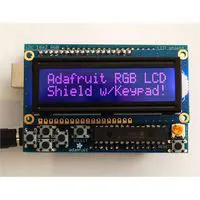RGB LCD Shield Kit, 16x2 Character Display
×1LED (generic)
×2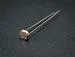Photo resistor
×1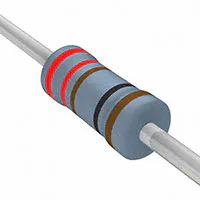Resistor 221 ohm
×2Resistor 10k ohm
×1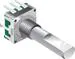Rotary Encoder with Push-Button
×1

## Necessary tools and machines

 Glove
 a small box

## About this project

Hello everyone,

we are Carlo Albani, Lorenzo Amaducci and Lorenzo Ferraiuolo from Liceo Scientifico A.Oriani, in Ravenna, Italy.

Our Arduino project is called "the Gesture Glove", a glove capable of substituting a computer mouse.

It has a gyroscope, that permits to move into the screen, a touch sensor, positioned on the forefinger, a rotaring encoder which replace the mouse wheel and a Calibrating Box that follow us during the calibration.

Firtly, we understand how the gyroscope works, and then we use it as a cursor.

The Construction Phase consisted in putting all the hardware in the glove, in the following order:

1. Gyroscope, 2. The battery, 3. Touch sensor, 4. Wireless sensor, 5. The rotaring encoder.

The Battery assemble is shown here:

That's the complete Gesture glove:

After this we builded the Calibrating Box, which is essential since the gyroscope need to set, and for doing it, he needs a flat surface.

The Calibrating Box has an LCD Display that gives you the necessary information, it also had two leds:

the first is red and is turning on during the calibration (you have 10 seconds for positioning the glove, in this period of time the led flashes), then when it ends, a green lights turn on and you can start to use the Gesture Glove.

Here's the link for our project's official account, you can go and check the final test, we would really appreciate you stopping by!

We sincerely hope you enjoyed our work and the effort we put in, have a nice day and let us know if you have any question!

INSTAGRAM LINK: https://www.instagram.com/gesture_glove/?hl=it

## Code

##### GestureGlove.inoC/C++
```#include "Wire.h"
#include "I2Cdev.h"
#include "MPU6050_6Axis_MotionApps20.h"
#include "Calibration_func.h"
#include "MPU6050_data_func.h"

float ypr;
String testo;
bool calibrate=false;
int lastMSB=0,lastLSB=0;
long lastencoderValue=0;
const uint8_t encoderPin1=2,encoderPin2=3,touch_pin=8;

volatile int lastEncoded=0;
volatile long int encoderValue=0;

MPU6050 mpu(0x68);

void setup(){

Serial.begin(115200);
Wire.begin();
TWBR=24;
/*
mpu.initialize();
mpu6050_calibration(mpu);
mpu.dmpInitialize();
mpu.setDMPEnabled(true);
attachInterrupt(0,dmpDataReady,RISING);
packetSize=mpu.dmpGetFIFOPacketSize();
*/
pinMode(touch_pin,INPUT);
pinMode(encoderPin1,INPUT_PULLUP);
pinMode(encoderPin2,INPUT_PULLUP);
attachInterrupt(0,updateEncoder,CHANGE);
attachInterrupt(1,updateEncoder,CHANGE);

}

void loop(){

if(!calibrate){
testo="";
while(Serial.available()) testo+=(char)Serial.read();
if(testo!=""){
mpu6050_calibration(mpu);
mpu.dmpInitialize();
mpu.setDMPEnabled(true);
attachInterrupt(0,dmpDataReady,RISING);
packetSize=mpu.dmpGetFIFOPacketSize();
calibrate=true;
}
}else{
get_data(mpu,ypr);
Serial.print(ypr*180/M_PI);
Serial.print(";");
Serial.print(ypr*180/M_PI);
Serial.print(";");
Serial.print(ypr*180/M_PI);
Serial.print(";");
Serial.print(digitalRead(touch_pin));
Serial.print(";");
Serial.println(encoderValue);
}

}

void updateEncoder(){

int MSB=digitalRead(encoderPin1),LSB=digitalRead(encoderPin2),encoded=(MSB<<1)|LSB,sum=(lastEncoded<<2)|encoded;

if(sum==0b1101||sum==0b0100||sum==0b0010||sum==0b1011) encoderValue++;
if(sum==0b1110||sum==0b0111||sum==0b0001||sum==0b1000) encoderValue--;
lastEncoded=encoded;

}
```
##### CalibratingBox.inoC/C++
```#include<LiquidCrystal.h>

int valore;
String testo;
bool calibrated=false,calibrating=false;
uint8_t red_led=3,green_led=2,photo_resistor=A4;

LiquidCrystal lcd(7,8,9,10,11,12);

void setup(){

Serial.begin(115200);
lcd.begin(16,2);
pinMode(photo_resistor,INPUT);
pinMode(green_led,OUTPUT);
pinMode(red_led,OUTPUT);
digitalWrite(green_led,LOW);
digitalWrite(red_led,LOW);

}
void loop(){

valore=analogRead(photo_resistor);
if(!calibrating&&!calibrated&&valore<50){
lampeggio(red_led,10,500);
digitalWrite(red_led,HIGH);
Serial.println("S");
calibrating=true;
}else if(calibrated&&valore>300){
digitalWrite(green_led,LOW);
digitalWrite(red_led,LOW);
calibrating=false;
}if(calibrating){
testo="";
while(Serial.available()) testo+=(char)Serial.read();
if(testo!="") lcd.clear();
if(testo=="FINISHED"){
digitalWrite(green_led,HIGH);
digitalWrite(red_led,LOW);
calibrated=true;
}
lcd.setCursor(0,0);
lcd.print(testo);
}

}

void lampeggio(uint8_t led,int n,int millisec){

for(int i=0;i<n;++i){
digitalWrite(led,HIGH);
delay(millisec);
digitalWrite(led,LOW);
delay(millisec);
}
}
```
##### serial_mouse.pyPython
```import pyautogui
import serial
import time

vel_sens=0.1

def strTofloat(data):

try:
num=data.split('.')
return int(num)+int(num)/10**len(num)
except IndexError:
return int(data)

arduino=serial.Serial("COM4",115200,timeout=0.05)
arduino2=serial.Serial("COM3",115200,timeout=0.05)
pyautogui.PAUSE=0
pyautogui.FAILSAFE=False
data=0; k=0;
x=0; y=0; z=0; t=0; s=0; x0=0; y0=0; z0=0; t0=0; s0=0;
calibrated=False
calibrating=False

arduino.flushInput()
arduino2.flushOutput()
while True:
if not calibrating and not calibrated:
data2=arduino2.readline()
if data2:
print(data2)
arduino.write(data2)
calibrating=True
else:
try:
data=arduino.readline()
x,y,z,t,s=[strTofloat(i) for i in str(data)[2:-5].split(';')]
except ValueError:
if(data):
arduino2.write(data)
print(data)
continue
try:
vy=y-y0
vz=z-z0
vx=x-x0
vs=s-s0
if calibrated:
if(t): pyautogui.mouseDown()
elif(t<t0): pyautogui.mouseUp()
pyautogui.scroll(vs*20)
pyautogui.moveRel(-vy*vel_sens,-vz*vel_sens,duration=0)
elif k>0 and abs(vx)<0.5 and abs(vy)<0.5 and abs(vz)<0.5:
k+=1
if k==50:
x0,y0,z0=x,y,z
calibrated=True
calibrating=False
arduino2.write(b"FINISHED")
elif k==0:
x0,y0,z0=x,y,z
k=1
else: k=0
except Exception as i:
print(i)
t0=t
s0=s
arduino.close()
arduino2.close()
```
##### MPU6050_data_func.hC/C++
```uint8_t mpuIntStatus;   // holds actual interrupt status byte from MPU
uint16_t packetSize;    // expected DMP packet size (default is 42 bytes)
uint16_t fifoCount;     // count of all bytes currently in FIFO
uint8_t fifoBuffer; // FIFO storage buffer
Quaternion q;           // [w, x, y, z]         quaternion container
VectorFloat gravity;    // [x, y, z]            gravity vector
uint8_t teapotPacket = { '\$', 0x02, 0,0, 0,0, 0,0, 0,0, 0x00, 0x00, '\r', '\n' };
volatile bool mpuInterrupt = false;     // indicates whether MPU interrupt pin has gone high

void dmpDataReady(){

mpuInterrupt=true;

}

void get_data(MPU6050 mpu,float ypr){

//ypr=0.0f; ypr=0.0f; ypr=0.0f;
mpuIntStatus=mpu.getIntStatus();
fifoCount=mpu.getFIFOCount();
if(mpuIntStatus&0x02){
while(fifoCount<packetSize) fifoCount=mpu.getFIFOCount();
mpu.getFIFOBytes(fifoBuffer,packetSize);
fifoCount-=packetSize;
mpu.dmpGetQuaternion(&q,fifoBuffer);
mpu.dmpGetGravity(&gravity,&q);
mpu.dmpGetYawPitchRoll(ypr,&q,&gravity);
}

}
```
##### Calibration_func.hC/C++
```#define BUFFERSIZE 1000     //Amount of readings used to average, make it higher to get more precision but sketch will be slower  (default:1000)
#define ACEL_DEADZONE 8     //Acelerometer error allowed, make it lower to get more precision, but sketch may not converge  (default:8)
#define GIRO_DEADZONE 1     //Giro error allowed, make it lower to get more precision, but sketch may not converge  (default:1)

void calibration(MPU6050);
void meansensors(MPU6050);

int16_t ax,ay,az,gx,gy,gz;
int mean_ax,mean_ay,mean_az,mean_gx,mean_gy,mean_gz,ax_offset,ay_offset,az_offset,gx_offset,gy_offset,gz_offset;

void mpu6050_calibration(MPU6050 accelgyro){

accelgyro.initialize();
Serial.println("Calibration");
delay(3000);
Serial.println(accelgyro.testConnection()?"Success":"Epic Fail?");
delay(1000);
accelgyro.setXAccelOffset(0);
accelgyro.setYAccelOffset(0);
accelgyro.setZAccelOffset(0);
accelgyro.setXGyroOffset(0);
accelgyro.setYGyroOffset(0);
accelgyro.setZGyroOffset(0);
delay(1000);
Serial.println("Reading sensors");
meansensors(accelgyro);
delay(1000);
Serial.println("Calculating offsets?");
calibration(accelgyro);
delay(1000);
meansensors(accelgyro);
Serial.print("Just a minute?");
accelgyro.setXGyroOffset(gx_offset);
accelgyro.setYGyroOffset(gy_offset);
accelgyro.setZGyroOffset(gz_offset);
accelgyro.setZAccelOffset(az_offset);

}

void meansensors(MPU6050 accelgyro){

long i=0,buff_ax=0,buff_ay=0,buff_az=0,buff_gx=0,buff_gy=0,buff_gz=0;

do{
accelgyro.getMotion6(&ax, &ay, &az, &gx, &gy, &gz); // read raw accel/gyro measurements from device
if (i>100 && i<=BUFFERSIZE+100){ //First 100 measures are discarded
buff_ax=buff_ax+ax;
buff_ay=buff_ay+ay;
buff_az=buff_az+az;
buff_gx=buff_gx+gx;
buff_gy=buff_gy+gy;
buff_gz=buff_gz+gz;
}if (i==BUFFERSIZE+100){
mean_ax=buff_ax/BUFFERSIZE;
mean_ay=buff_ay/BUFFERSIZE;
mean_az=buff_az/BUFFERSIZE;
mean_gx=buff_gx/BUFFERSIZE;
mean_gy=buff_gy/BUFFERSIZE;
mean_gz=buff_gz/BUFFERSIZE;
}
delay(2); //Needed so we don't get repeated measures
}while(++i<=BUFFERSIZE+100);

}

void calibration(MPU6050 accelgyro){

int ready;

ax_offset=-mean_ax/8;
ay_offset=-mean_ay/8;
az_offset=(16384-mean_az)/8;
gx_offset=-mean_gx/4;
gy_offset=-mean_gy/4;
gz_offset=-mean_gz/4;
do{
ready=0;
accelgyro.setXAccelOffset(ax_offset);
accelgyro.setYAccelOffset(ay_offset);
accelgyro.setZAccelOffset(az_offset);
accelgyro.setXGyroOffset(gx_offset);
accelgyro.setYGyroOffset(gy_offset);
accelgyro.setZGyroOffset(gz_offset);
meansensors(accelgyro);
Serial.println("...");
if (abs(mean_ax)<=ACEL_DEADZONE) ready++;
else ax_offset=ax_offset-mean_ax/ACEL_DEADZONE;
if (abs(mean_ay)<=ACEL_DEADZONE) ready++;
else ay_offset=ay_offset-mean_ay/ACEL_DEADZONE;
if (abs(16384-mean_az)<=ACEL_DEADZONE) ready++;
else az_offset=az_offset+(16384-mean_az)/ACEL_DEADZONE;
if (abs(mean_gx)<=GIRO_DEADZONE) ready++;
else gx_offset=gx_offset-mean_gx/(GIRO_DEADZONE+1);
if (abs(mean_gy)<=GIRO_DEADZONE) ready++;
else gy_offset=gy_offset-mean_gy/(GIRO_DEADZONE+1);
if (abs(mean_gz)<=GIRO_DEADZONE) ready++;
else gz_offset=gz_offset-mean_gz/(GIRO_DEADZONE+1);
}while(ready!=6);

}
```

## Schematics

we didn't put the touch sensor because we can't find it in Fritzing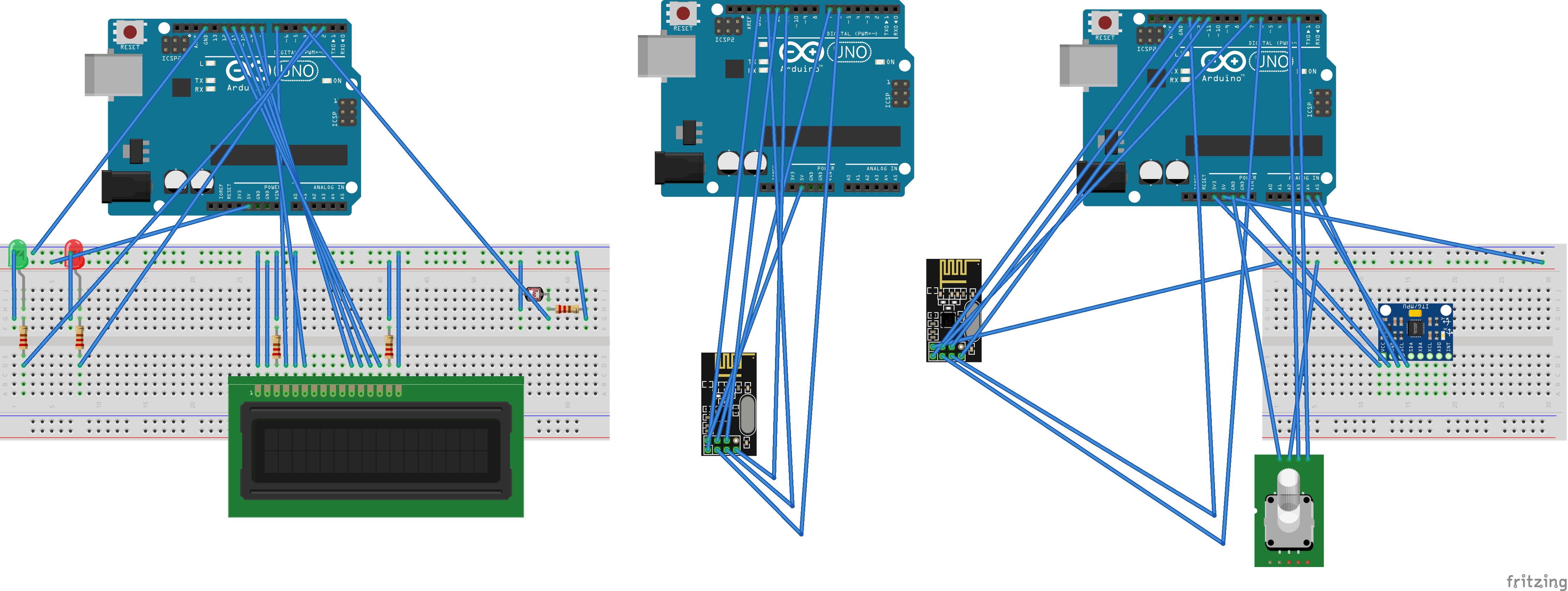## Comments

#### Created by

Albani Carlo Amaducci Lorenzo Ferraiuolo Lorenzo

Are you Albani Carlo Amaducci Lorenzo Ferraiuolo Lorenzo? Claim this project and add it to your profile.

#### Published on

November 18, 2019

#### Members who respect this project

See similar projects
you might like

#### Gesture Drive: Accelerate with Freedom

Project tutorial by Shahariar

• 4,543 views
• 0 comments
• 13 respects

#### Gesture Recognition Using Accelerometer and ESP

Project tutorial by mellis

• 53,966 views
• 27 comments
• 90 respects

• 7,696 views
• 2 comments
• 10 respects

#### Remote Lamp

Project tutorial by Kutluhan Aktar

• 4,447 views
• 0 comments
• 9 respects

#### Laser, Robe, Star Wars?! - Versalume + Adafruit Gemma

Project tutorial by Kitty Yeung

• 3,696 views
• 2 comments
• 11 respects

#### DIY Glove Controlled Robotic Hand

Project showcase by Laurence Lai

• 692 views
• 1 comment
• 7 respects